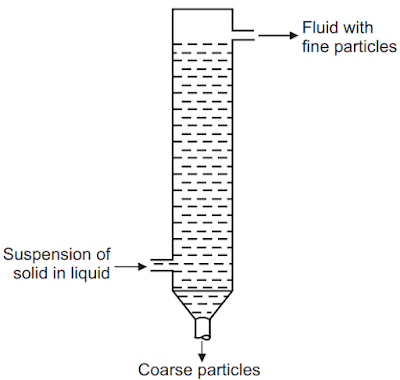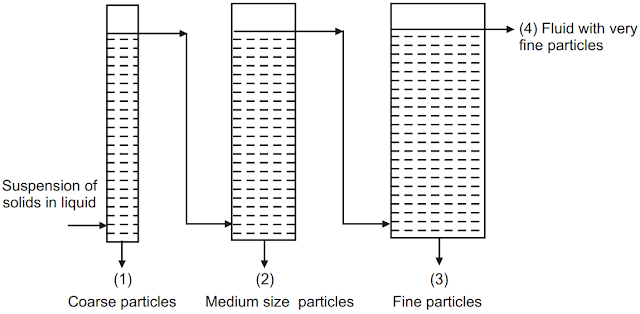# Elutriation Tank – Principle, Construction, Working, and More

Elutriation Tank: An elutriator is a simple device that can separate particles into two or more groups. Elutriation depends on the movement of a fluid against the direction of sedimentation of the particles. Elutriation is based on particle size, shape, and density. The air elutriator is mainly used for particles smaller than 1 µm. The smaller or lighter particles rise to the top (overflow) because their terminal sedimentation velocities are lower than the velocity of the rising fluid. The terminal velocity of any particle in any medium can be calculated using Stokes' law if the particle's Reynolds number is below 0.2. In elutriator particles, separation is based upon densities. The heavier particles settle due to gravitational force.

## Principle of Elutriation Tank

In the process of elutriation, particles falling in a rising fluid can be classified into two sizes. When the fluid in a sorting column is rising with a certain velocity, the particles having terminal velocities higher than this velocity settle at the bottom of the sorting column, and the particles with lower terminal velocities are lifted to the top of the sorting column and are carried away to the next tube. The terminal velocities of the particles falling in a fluid can be calculated using the Stokes' law equation for Reynolds number.

R_e=\frac{2r\times V_m\times(\rho-\rho_o)}\eta ...(1)

V_m=\frac{2r^2\times g\times(\rho-\rho_o)}{9\eta} ...(2)

Where, ρ = specific gravity of the particle, ρo specific gravity of the fluid medium, r is the radius of the particle, η = viscosity of the medium, Vm = terminal velocity of the particle, and g = gravitational acceleration.

In the elutriation, when the volumetric flow rate of rising fluid is constant, the velocity of the rising fluid in the columns depends on their diameters. The narrow diameter column gives high velocity and the one with wide diameter gives low fluid velocity. Higher velocities of the rising medium allow coarser particles to settle while lower velocities allow finer particles to settle. Various size classes of particles can be obtained when the sample is separated into columns of increasing diameters connected in series. The upper size limit of the particles follows Stokes' law.

## Construction of Elutriation Tank

For the gravitational system, the apparatus consists simply of a vertical column with an inlet near the bottom for the suspension, an outlet at the base for coarse particles, and an overflow near the top for fluid, Fig.1. One column will give a single separation into two fractions, but it must be remembered that this will not give a clear cut separation, since there is a velocity gradient across the tube, resulting in the separation of particles of different sizes according to the distance from the wall. If more than one fraction is required, several tubes of increasing area of the cross-section can be connected in series, Fig.2.Fig.1: A Schematic of Simple Elutriator

With the same overall flow rate, the velocity will decrease in succeeding tubes as the area of cross-section increases, giving several fractions.Fig.2: Multi-Stage Elutriator: (1) to (4) is fractions of decreasing particle size

## Working of Elutriation Tank

The material whose particles are to be separated is first levigated and the paste is transferred to the elutriation tank. A large amount of water is added to the tank to make independent particle settling. The contents in the tank are stirred to obtain uniform particle distribution. If left aside undisturbed coarse particles settle at the bottom whereas small size particles remain suspended in a liquid. These fines can be transferred to the next elutriator in connection wherein a similar process of separation takes place to obtain further fractions of fines.

1. The process is continuous.
2. The separation is quicker than with sedimentation.
3. It has the feasibility to add many columns based upon the fractions required.
4. It needs no skilled operators.
5. It is a fast process than sedimentation.

Make sure you also check our other amazing Article on : Bag filter